##### Geometry: Place-Out Exam

We want to make sure you take classes that aren't too easy for you. This course will have new things for you to learn if you get less than 70% of this test.

Time Left:

1:00:00

1.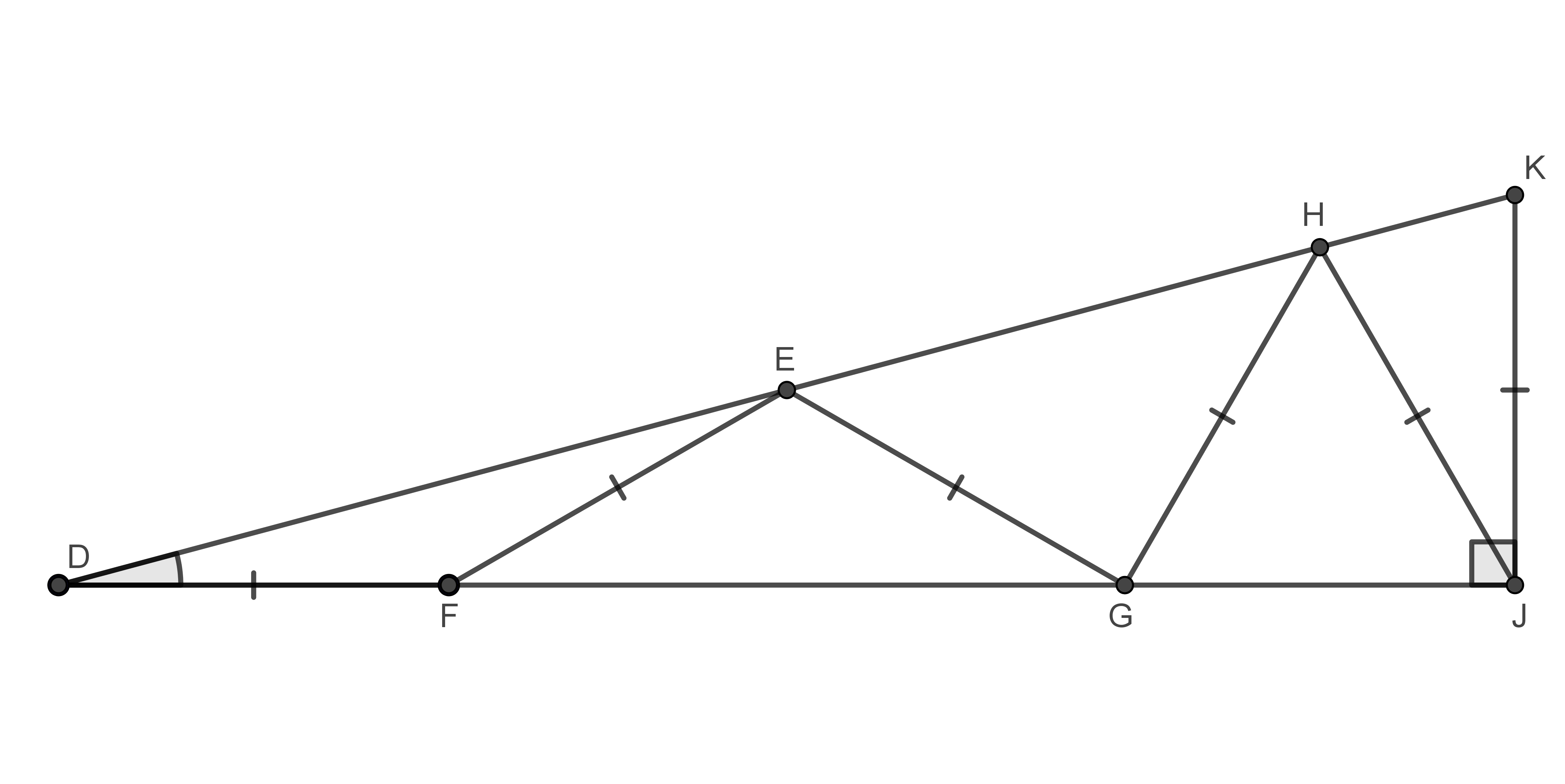What is the measure of $$\angle D?$$ Express your answer in degrees rounded to the nearest whole degree.

If your answer is $$31.4^{\circ},$$ you would enter: $$31$$

2.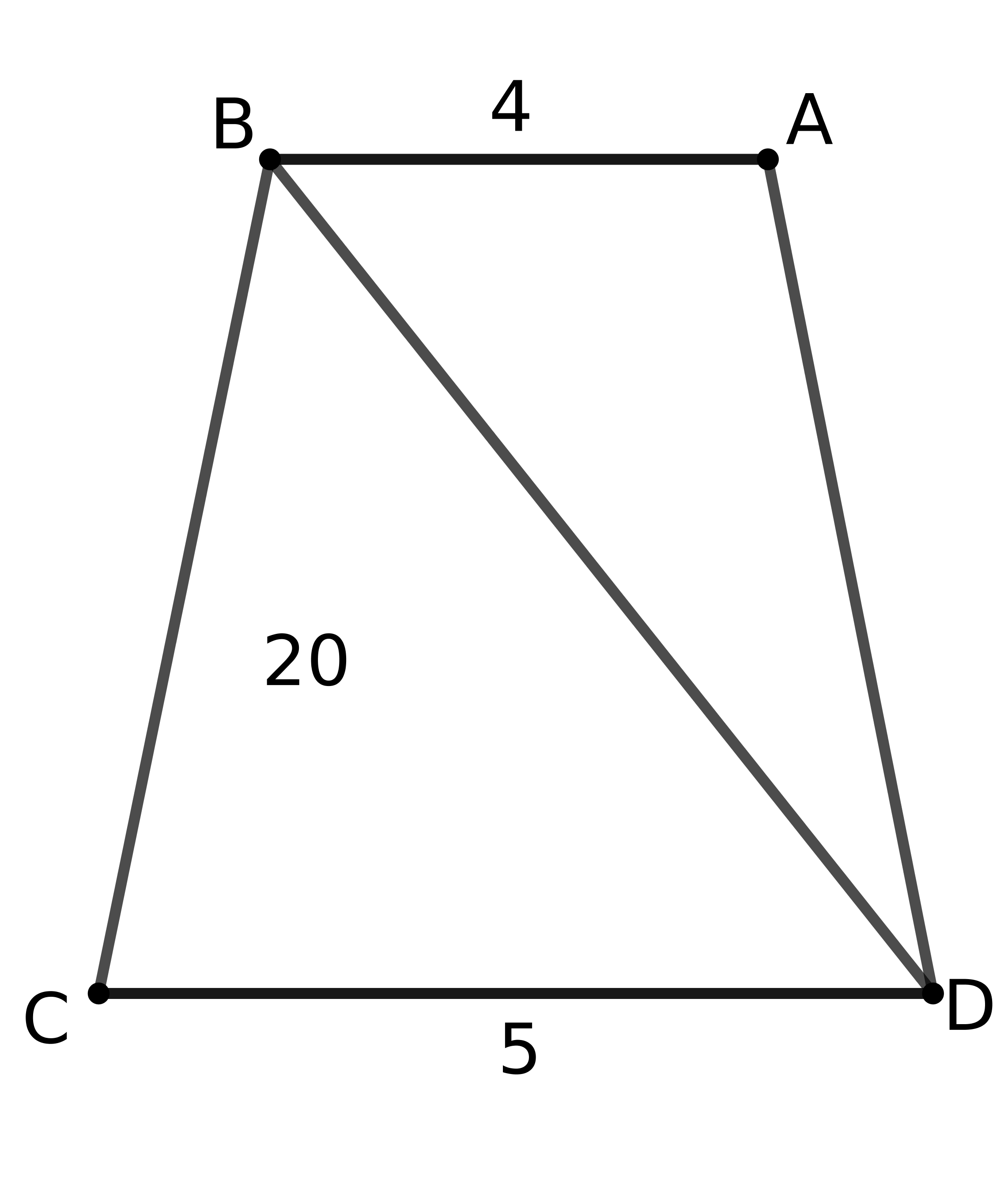Sides $$AB$$ and $$CD$$ are parallel. The area of $$\bigtriangleup BCD$$ is $$20.$$ What is the area of $$\bigtriangleup ABD?$$ (The diagram is not drawn to scale.)

3.

Suppose $$B$$ and $$C$$ are endpoints of a diameter of a circle. If $$A$$ is another point on this circle, and $$AB = 12$$ and $$AC = 16,$$ what is the radius of the circle?

4.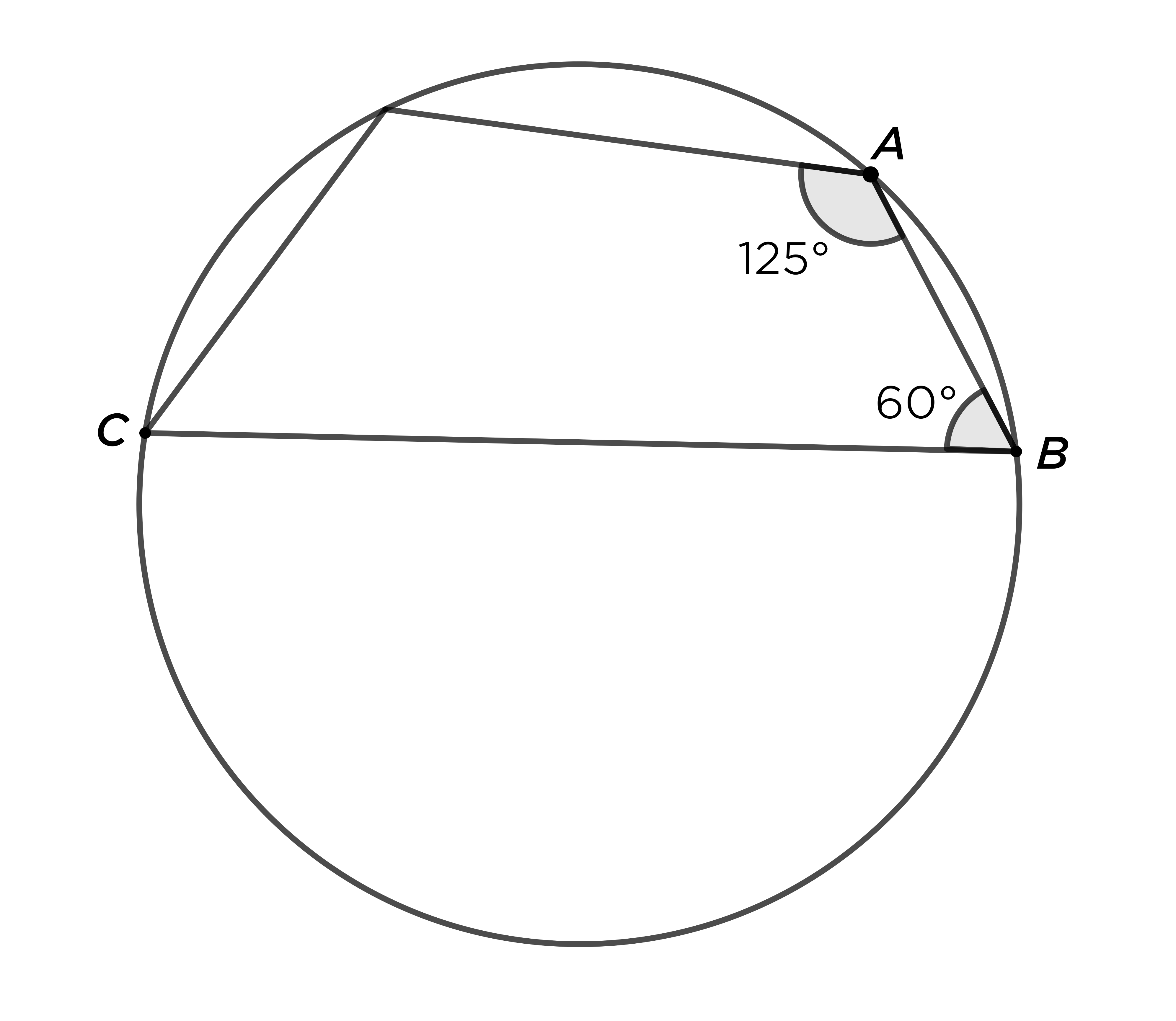If the four corners of this quadrilateral are all on the circle, and $$\angle A = 125^\circ$$ and $$\angle B = 60^\circ,$$ how many degrees are in $$\angle C?$$

5.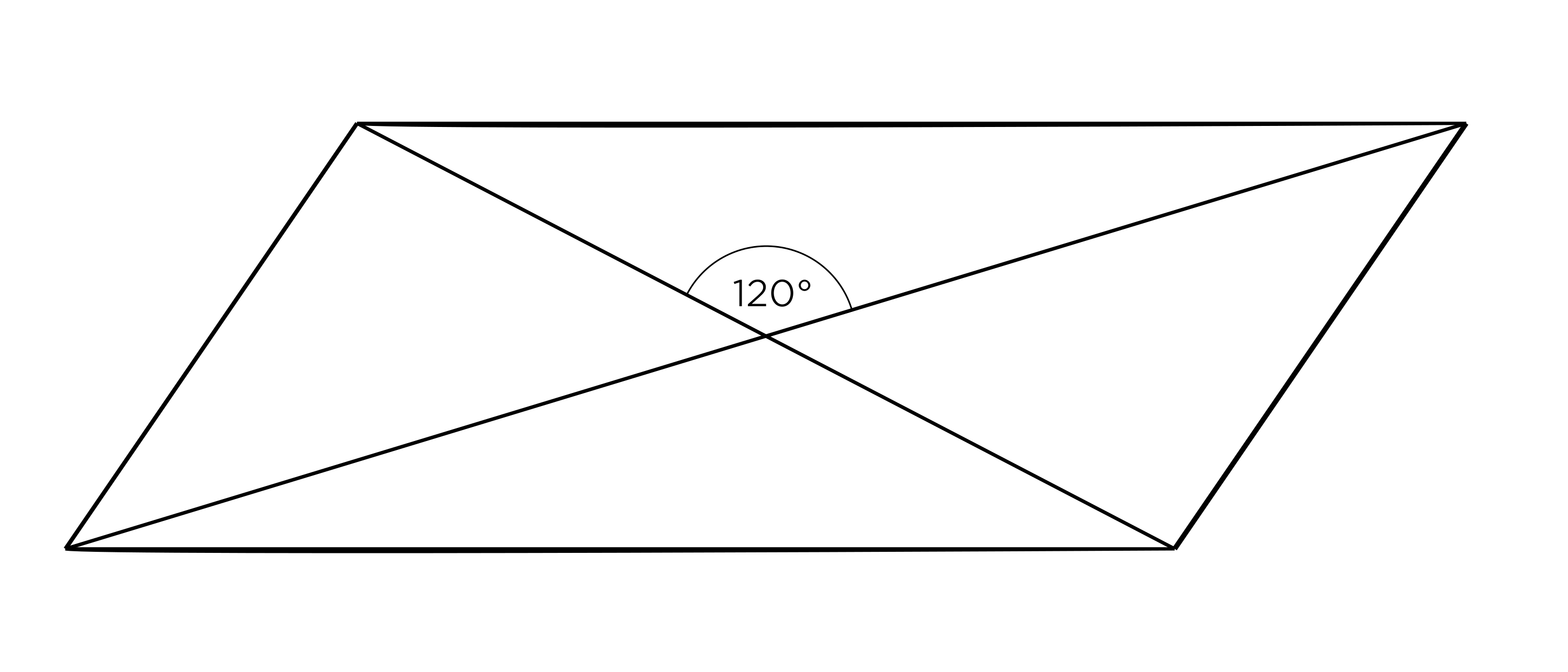Let $$a$$ be the area of this parallelogram with diagonals $$8$$ and $$4.$$ What is $$a^2?$$

6.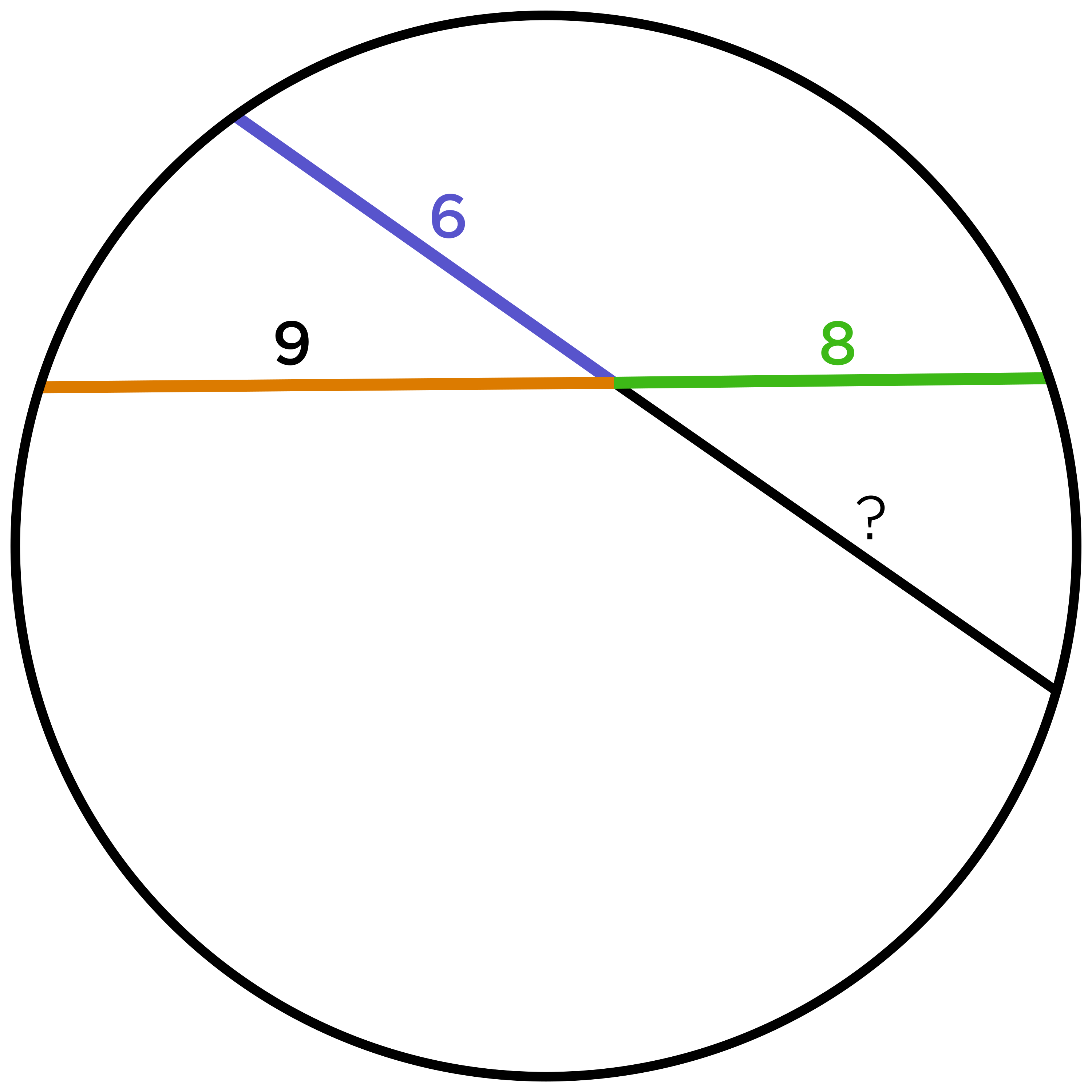In a circle with radius $$12$$, two chords intersect, creating segments of length $$6,$$ $$8,$$ and $$9,$$ as shown. What is the length of the missing segment?

7.

How many different triangles with integer side lengths have a perimeter of $$8?$$

8.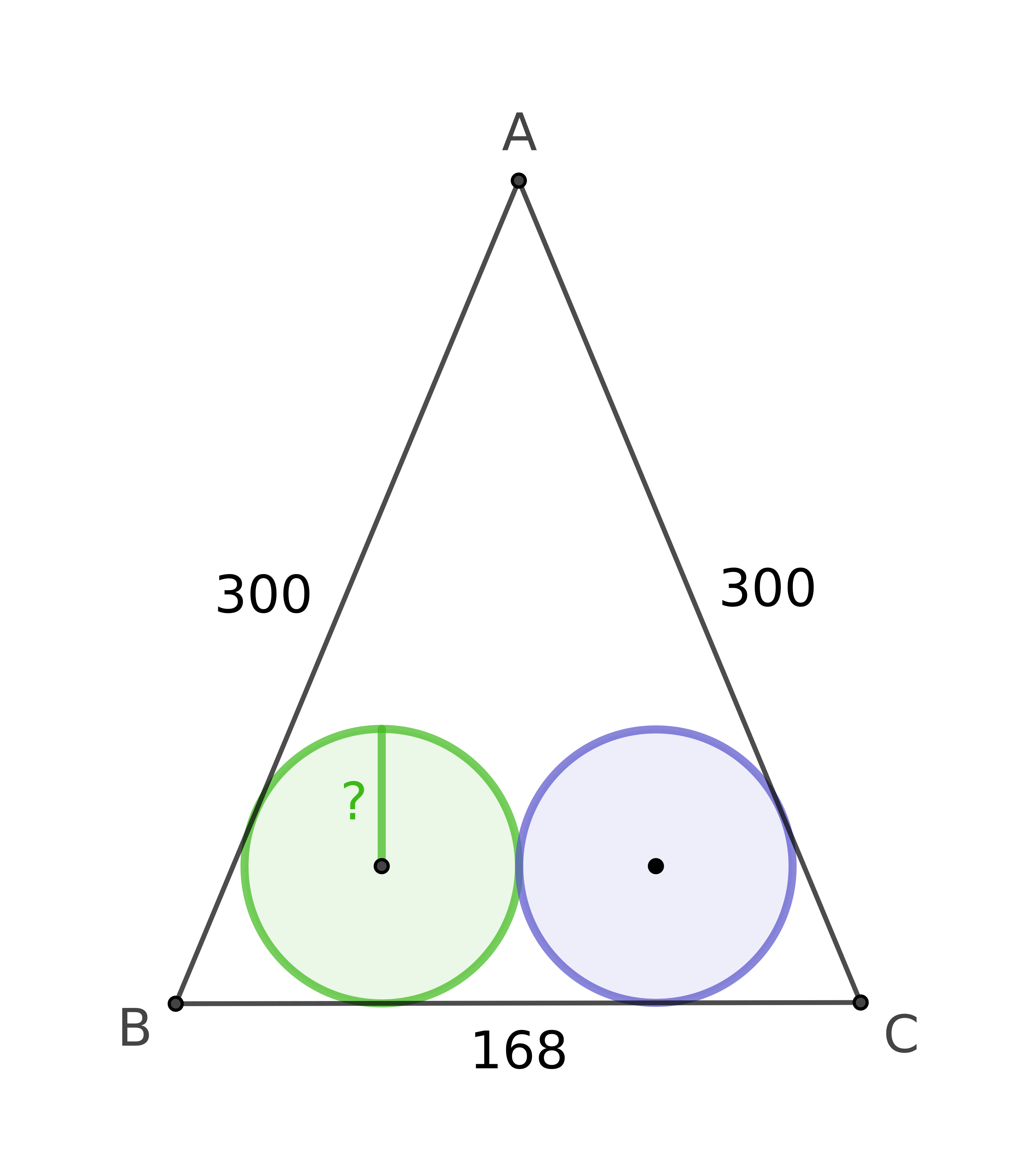An isosceles triangle has sides of $$AB = 300,$$ $$AC = 300,$$ and $$BC = 168.$$ There are two inscribed circles with the same radius, both internally tangent to the triangle along $$\overline{BC}.$$ What is the length of the radius of the circles?

9.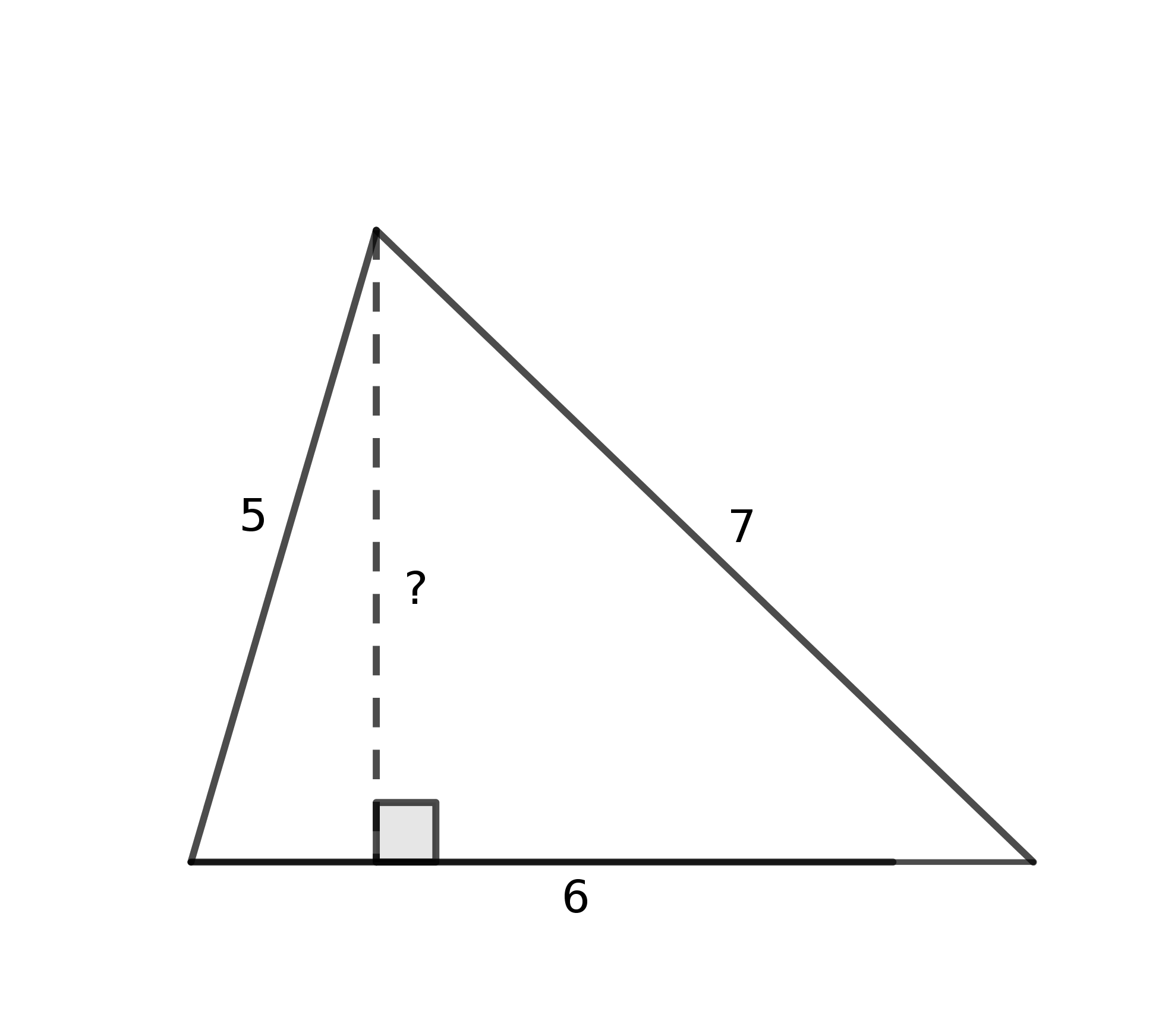What is the square of the height of this triangle?

10.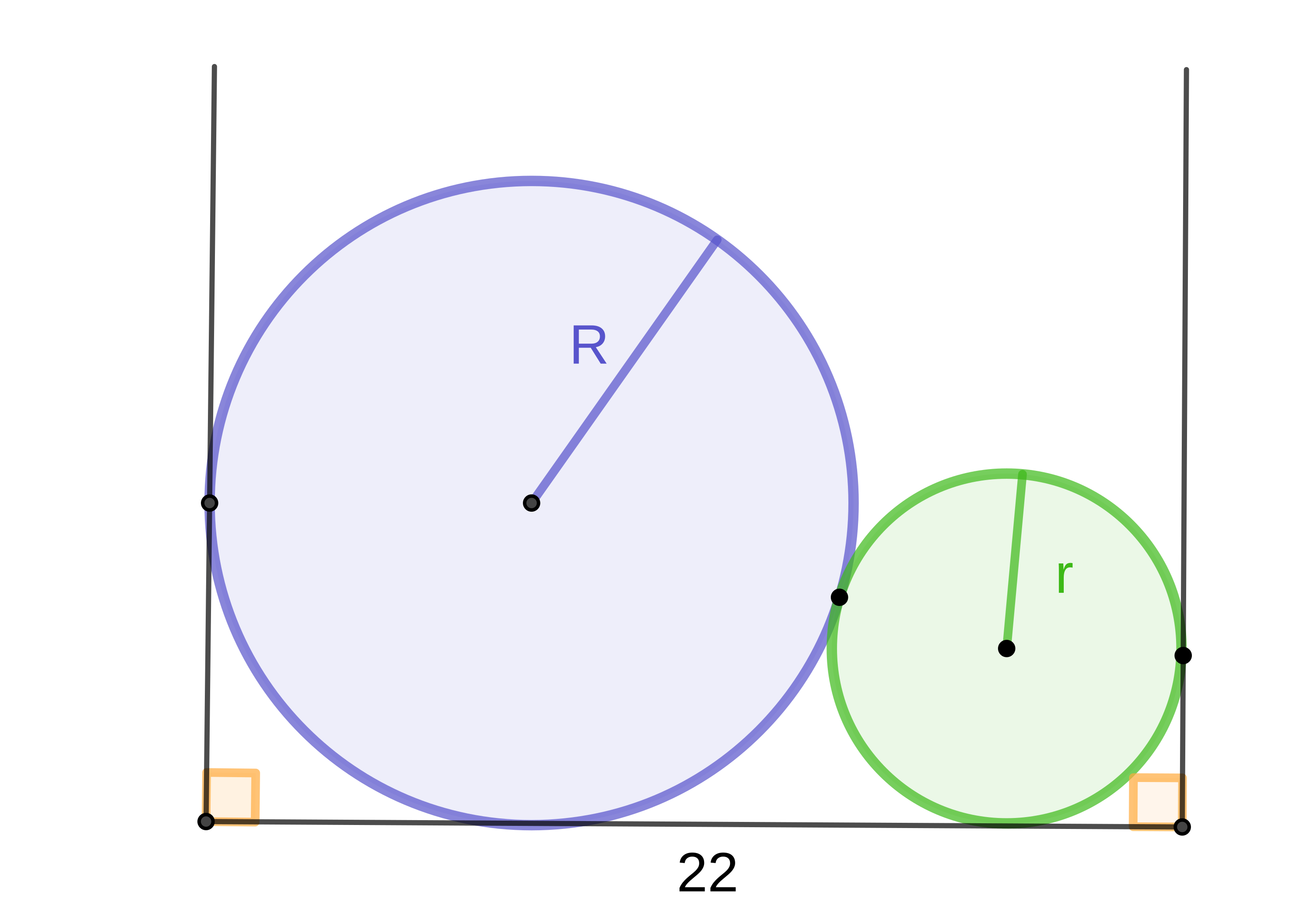Two balls fit snugly between two walls that are $$22$$ inches apart. The sum of the radii of the balls is $$12.$$ What is the product of the two radii?